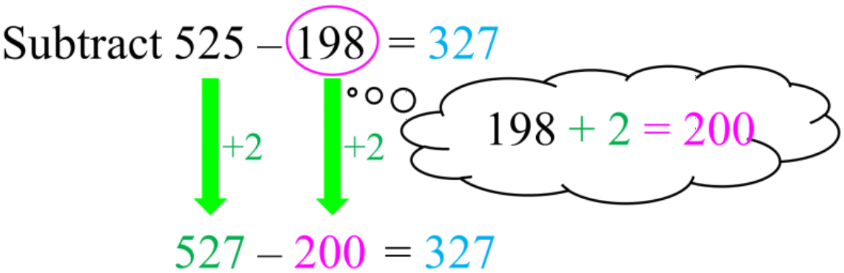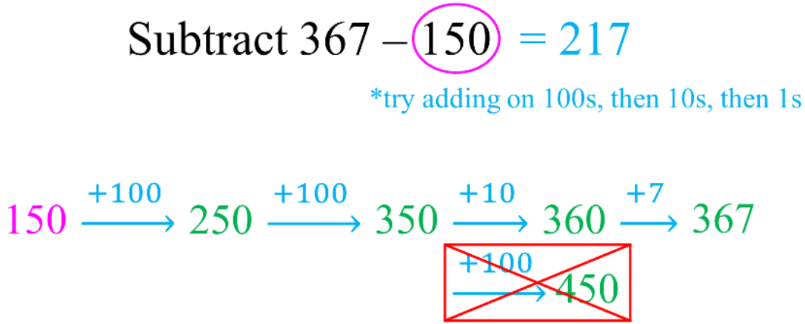# Subtraction strategies#### All in One Place

Everything you need for better grades in university, high school and elementary.#### Learn with Ease

Made in Canada with help for all provincial curriculums, so you can study in confidence.#### Instant and Unlimited Help

0/4
##### Intros
###### Lessons
1. Introduction to Subtraction Strategies:
2. Mental math and differences of 10
3. Subtraction strategy #1: friendly number with adjustment
4. Subtraction strategy #2: friendly number by adding to both numbers
5. Subtraction strategy #3: counting on from the first number
0/19
##### Examples
###### Lessons
1. Subtracting by breaking up the subtrahend
Subtract by breaking up the number being taken away.
Fill in the blanks by following the example: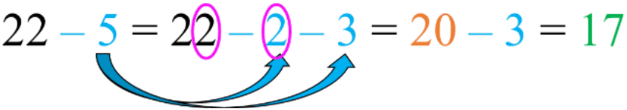1. 57 - 9 = 57 - 7 - ____ = 50 - ____ = ____
2. 64 - 7 = 64 - ____ - ____ = 60 - ____ = ____
3. 38 - 9 = 38 - ____ - ____ = 30 - ____ = ____
4. 92 - 8 = 92 - ____ - ____ = ____ - _____ = _____
2. Subtracting by 9 strategy
Subtract 9 by: subtracting 10 first, then putting 1 back
1. 17 - 9 = ____
17 - 10 = ____ + 1 = ____
2. 42 - 9 = ____
42 - 10 = ____ + 1 = ____
3. 35 - 9 = ____
35 - 10 = ____ + 1 = ____
3. Subtracting by 8 strategy
Subtract 8 by: subtracting 10 first, then putting 2 back
1. 15 - 8 = ____
15 - 10 = ____ + 2 = ____
2. 63 - 8 = ____
63 - 10 = ____ + 2 = ____
3. 47 - 8 = ____
47 - 10 = ____ + 2 = ____
4. Subtracting using friendly numbers with adjustment
Subtract by changing one number into a "friendly" number and adjust. Fill in the blanks.
1.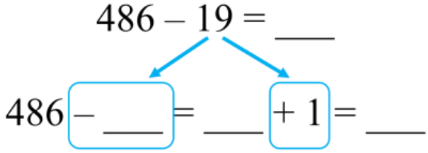2.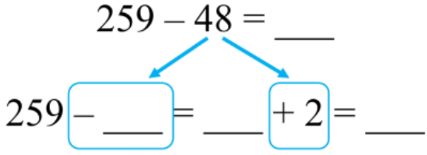3.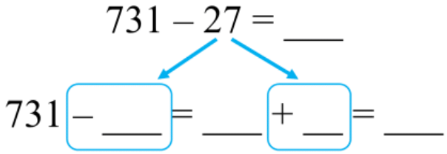5. Subtraction strategy: make friendly numbers by adding to both numbers
Subtract: make "friendly" numbers by adding to both numbers. Fill in the blanks.
1.2.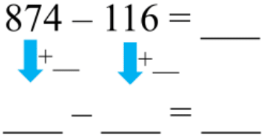3.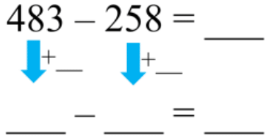6. Subtraction strategy: counting on from the second number
Subtract by counting on from the second number. Show your work.
1. 573 - 252 = ____
2. 408 - 126 = ____
3. 847 - 639 = ____
0%
##### Practice
###### Topic Notes

In this lesson, we will learn:

• The subtraction strategy of: (1) using a friendly number with adjustment, (2) adding to both numbers to create a friendly number, and (3) counting on from the first number (subtrahend)
• Understanding subtracting by 9

Notes:

• Method 1: change into a "friendly" number then adjust (by adding)
• Round one the number being taken away to the nearest multiple of 10